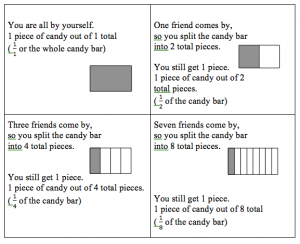# Ordering and Comparing Fractions

When students are asked to order and compare fractions, they almost always start by finding common denominators. This strategy is based on rote memorization and leads to little or no true understanding of fractions (and can be utterly frustrating!). Students cannot visualize the fractions. This article explains how to help your students compare and order fractions using reasoning skills, not math formulas.

There are three steps outlined below. Each step should be introduced separately, practiced and then combined with the steps learned previously.

Step 1 – Use benchmarks – Using benchmarks of 0, 1 and greater than 1 (improper fractions) help students get a general idea of the size of the fraction.

Example – Put the following fractions in order from least to greatest:

Encourage students to find those fractions that are equivalent to 0, 1 and greater than 1 first. Then identify if any of the fractions are exactly  Compare the numerator and denominators on the remaining fractions to determine if they are less than  or more than  Try to relate the fraction to real life examples. (“If I received 11 out of 12 on a test, did I get more than half the questions correct or fewer than half the questions correct?”)

Provide a simple table for those students who have trouble organizing their work.

Step 2 – Use Common Denominators – Many students think ordering fractions with common denominators is even easier than using benchmarks. Since each fraction will have the same number of parts to make the total, comparing is easy. Again, present fractions in real life situations that allow students to visualize them. For example, if you took a math quiz worth 25 points, who would get more of the quiz correct: the student who gets 24 questions correct (  ) or the student who gets 13 questions correct (  )?

Step 3 – Use Common Numerators – This strategy is a bit more difficult for students to grasp. The use of fraction towers, fraction circles and/or drawings helps students grasp this concept.

When the numerators are the same, you are receiving the same number of pieces of the object. However, since the denominators are different, the whole will be cut into a different amount of pieces. For example, imagine you are eating a candy bar. You receive one piece (the numerator), no matter what. If you are all by yourself, you get the whole candy bar. Now imagine one of your friends comes by. You want to share the candy bar; so you split it into 2 pieces (in half). What happens to the size of your one piece as you share with more and more friends?For a pie version of this, Birmingham Learning Resources shows us:  http://www.bgfl.org/custom/resources_ftp/client_ftp/ks2/maths/fractions/numerators.htm

And: http://www.freemathhelp.com/numerator-denominator.html

And for more of our Fun Learning Math Games, you can visit here:

http://www.math-lessons.ca/activities/index.html